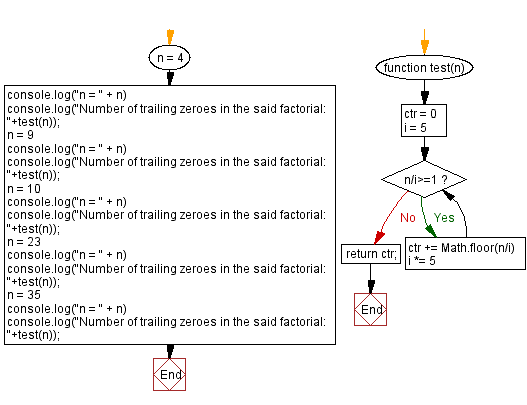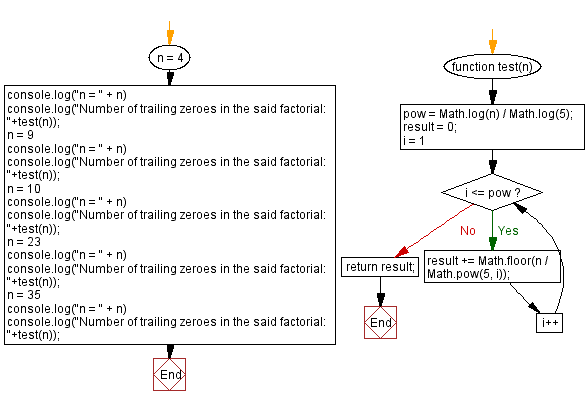# JavaScript: Number of trailing zeroes in a factorial

## JavaScript Math: Exercise-96 with Solution

Write a JavaScript program that calculates the factorial of a number and returns the number of trailing zeroes.

In mathematics, the factorial of a non-negative integer n, denoted by n!, is the product of all positive integers less than or equal to n. The factorial of n also equals the product of n with the next smaller factorial:
n! = n x (n-1) x (n-2) x (n-3)x.....x 3 x 2 x 1
= n x (n-1)!
For example 4! = 4 x 3! = 4 x 3 x 2 x 1 =24
The value of 0! is 1, according to the convention for an empty product.

Test Data:
(4) -> 0
(9) -> 1
(10) -> 2
(23) -> 4
(35) -> 8

Sample Solution-1:

HTML Code:

``````<!DOCTYPE html>
<html>
<meta charset="utf-8">
<title>JavaScript program to Number of trailing zeroes in a factorial</title>
<body>

</body>
</html>
```
```

JavaScript Code:

``````function test(n) {
ctr = 0
i = 5
while (n/i>=1){
ctr += Math.floor(n/i)
i *= 5
}
return ctr;
};
n = 4
console.log("n = " + n)
console.log("Number of trailing zeroes in the said factorial: "+test(n));
n = 9
console.log("n = " + n)
console.log("Number of trailing zeroes in the said factorial: "+test(n));
n = 10
console.log("n = " + n)
console.log("Number of trailing zeroes in the said factorial: "+test(n));
n = 23
console.log("n = " + n)
console.log("Number of trailing zeroes in the said factorial: "+test(n));
n = 35
console.log("n = " + n)
console.log("Number of trailing zeroes in the said factorial: "+test(n));
```
```

Sample Output:

```n = 4
Number of trailing zeroes in the said factorial: 0
n = 9
Number of trailing zeroes in the said factorial: 1
n = 10
Number of trailing zeroes in the said factorial: 2
n = 23
Number of trailing zeroes in the said factorial: 4
n = 35
Number of trailing zeroes in the said factorial: 8
```

Flowchart:Live Demo:

See the Pen javascript-math-exercise-96 by w3resource (@w3resource) on CodePen.

Sample Solution-2:

JavaScript Code:

``````function test(n)
{
pow = Math.log(n) / Math.log(5);
result = 0;
for (i = 1; i <= pow; i++) {
result += Math.floor(n / Math.pow(5, i));
};
return result;
}

n = 4
console.log("n = " + n)
console.log("Number of trailing zeroes in the said factorial: "+test(n));
n = 9
console.log("n = " + n)
console.log("Number of trailing zeroes in the said factorial: "+test(n));
n = 10
console.log("n = " + n)
console.log("Number of trailing zeroes in the said factorial: "+test(n));
n = 23
console.log("n = " + n)
console.log("Number of trailing zeroes in the said factorial: "+test(n));
n = 35
console.log("n = " + n)
console.log("Number of trailing zeroes in the said factorial: "+test(n));
```
```

Sample Output:

```n = 4
Number of trailing zeroes in the said factorial: 0
n = 9
Number of trailing zeroes in the said factorial: 1
n = 10
Number of trailing zeroes in the said factorial: 2
n = 23
Number of trailing zeroes in the said factorial: 4
n = 35
Number of trailing zeroes in the said factorial: 8
```

Flowchart:Live Demo:

See the Pen javascript-math-exercise-96-1 by w3resource (@w3resource) on CodePen.

Improve this sample solution and post your code through Disqus

What is the difficulty level of this exercise?

Test your Programming skills with w3resource's quiz.

﻿